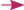## Formally verifying the complexity of OCaml programs with CFML -- interlude

This small interlude post is not about Coq nor CFML: I present a data structure from Okasaki’s Purely functional data structures, implemented in OCaml.

It comes as a preliminary explanation for an incoming formalization of it in CFML (see next post), including an complexity analysis!## Formally verifying the complexity of OCaml programs with CFML -- part 1

In a recent paper, Arthur Charguéraud and François Pottier present a formal proof of an OCaml implementation of Tarjan’s union-find algorithm. The proof covers two aspects: first, functional correctness (“the algorithm is correct”), but also asymptotic complexity. For example, one of the results of the paper is that the link function runs in $$O(α(n))$$ elementary steps, $$α$$ being the inverse of the Ackermann function.

Actually, the complexity results of the paper are not presented using the “big-O” notation, commonly used in asymptotic complexity proofs: the various constants are explicit and manipulated through the proof.

In these blog posts (current, interlude and final), I’ll describe a tentative extension of CFML, the Coq library François and Arthur used for their proof. Its goal is to enable asymptotic reasoning, big-O notations and such to be used to prove complexity of programs.